# Composite Carbonic Acid and Carbonate Kinetics

aqion
aqion
May. 28, 2016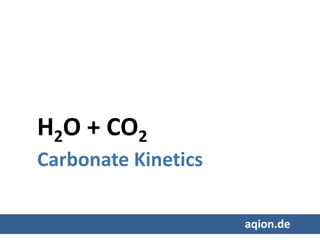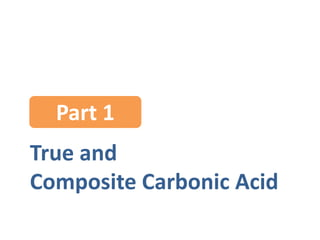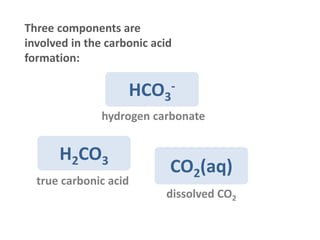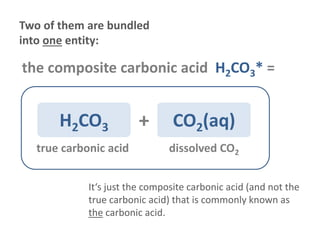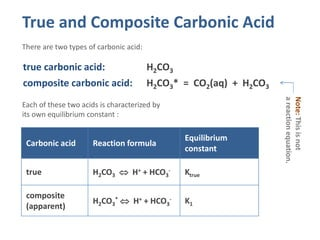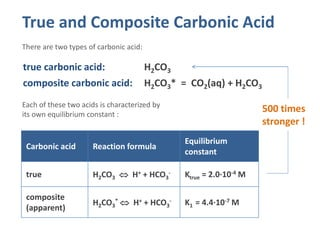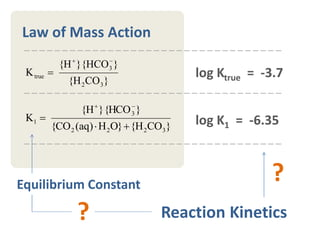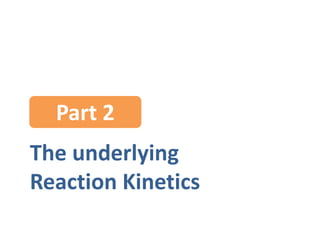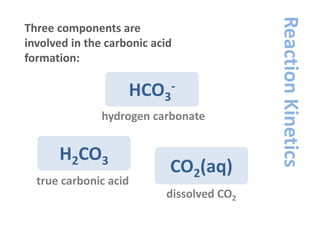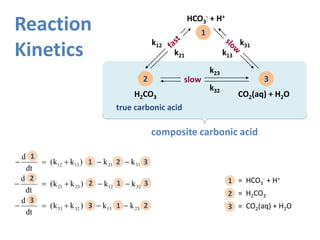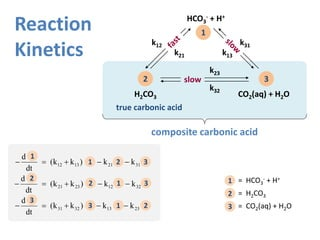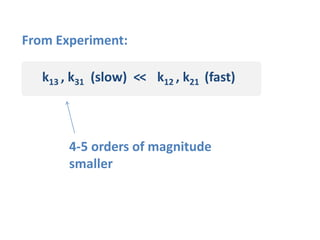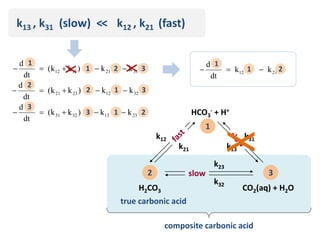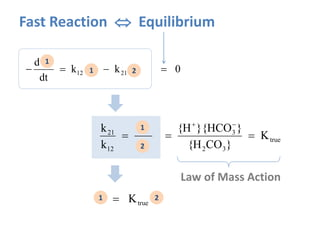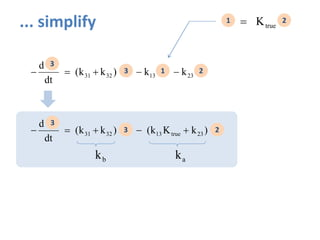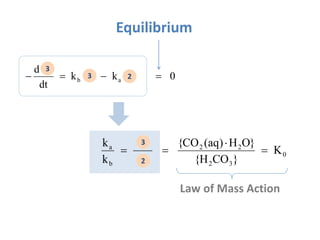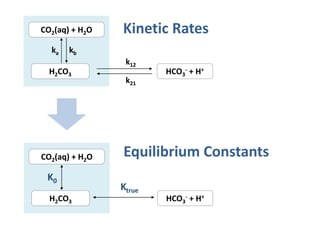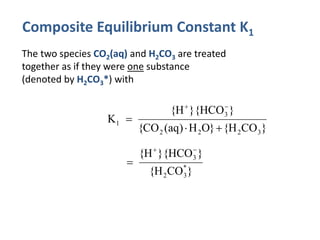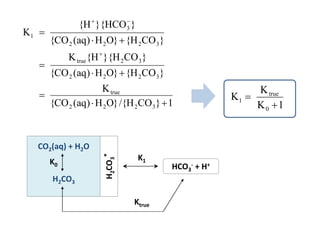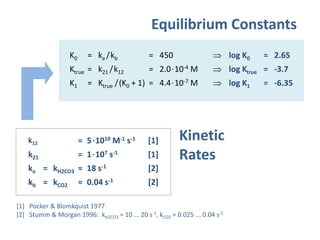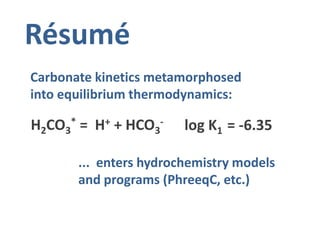1 of 22

### Composite Carbonic Acid and Carbonate Kinetics

1. H2O + CO2 Carbonate Kinetics aqion.de
2. True and Composite Carbonic Acid Part 1
3. Three components are involved in the carbonic acid formation: CO2(aq) H2CO3 HCO3 - true carbonic acid dissolved CO2 hydrogen carbonate
4. Two of them are bundled into one entity: CO2(aq)H2CO3 true carbonic acid dissolved CO2 the composite carbonic acid H2CO3* = + It‘s just the composite carbonic acid (and not the true carbonic acid) that is commonly known as the carbonic acid.
5. True and Composite Carbonic Acid true carbonic acid: H2CO3 composite carbonic acid: H2CO3* = CO2(aq) + H2CO3 There are two types of carbonic acid: Each of these two acids is characterized by its own equilibrium constant : Carbonic acid Reaction formula Equilibrium constant true H2CO3  H+ + HCO3 - Ktrue composite (apparent) H2CO3 *  H+ + HCO3 - K1 Note:Thisisnot areactionequation.
6. True and Composite Carbonic Acid true carbonic acid: H2CO3 composite carbonic acid: H2CO3* = CO2(aq) + H2CO3 There are two types of carbonic acid: Each of these two acids is characterized by its own equilibrium constant : Carbonic acid Reaction formula Equilibrium constant true H2CO3  H+ + HCO3 - Ktrue = 2.0·10-4 M composite (apparent) H2CO3 *  H+ + HCO3 - K1 = 4.4·10-7 M 500 times stronger !
7. }COH{}OH)aq(CO{ }HCO}{H{ K 3222 3 1    }COH{ }HCO}{H{ K 32 3 true   Law of Mass Action Reaction Kinetics log Ktrue = -3.7 log K1 = -6.35 ?Equilibrium Constant ?
8. The underlying Reaction Kinetics Part 2
9. Three components are involved in the carbonic acid formation: CO2(aq) H2CO3 HCO3 - true carbonic acid dissolved CO2 hydrogen carbonate ReactionKinetics
10. Reaction Kinetics CO2(aq) + H2O true carbonic acid HCO3 - + H+ H2CO3 1 2 3 k21 k13 k31 composite carbonic acid k23 k32 k12 slow 1 = HCO3 - + H+ 2 = H2CO3 3 = CO2(aq) + H2O2k1k3)kk( dt 3d 3k1k2)kk( dt 2d 3k2k1)kk( dt 1d 23133231 32122321 31211312    1 2 3 1 2 3 2 1 3 3 1 2 1 2 3
11. Reaction Kinetics CO2(aq) + H2O true carbonic acid HCO3 - + H+ H2CO3 1 2 3 k21 k13 k31 composite carbonic acid k23 k32 k12 slow 1 = HCO3 - + H+ 2 = H2CO3 3 = CO2(aq) + H2O2k1k3)kk( dt 3d 3k1k2)kk( dt 2d 3k2k1)kk( dt 1d 23133231 32122321 31211312    1 2 3 1 2 3 2 1 3 3 1 2 1 2 3
12. k13 , k31 (slow) << k12 , k21 (fast) 4-5 orders of magnitude smaller From Experiment:
13. k13 , k31 (slow) << k12 , k21 (fast) 2k1k3)kk( dt 3d 3k1k2)kk( dt 2d 3k2k1)kk( dt 1d 23133231 32122321 31211312    1 2 3 1 2 3 2 1 3 3 1 2 X X CO2(aq) + H2O true carbonic acid HCO3 - + H+ H2CO3 1 2 3 k21 k13 k31 composite carbonic acid k23 k32 k12 slow X 2k1k dt 1d 2112  1 1 2
14. Fast Reaction  Equilibrium 02k1k dt 1d 2112  1 1 2 true 32 3 12 21 K }COH{ }HCO}{H{ 2 1 k k   1 2 Law of Mass Action 2K1 true1 2
15. 2)kKk(3)kk( dt 3d 2k1k3)kk( dt 3d 23true133231 23133231   3 3 1 2 3 3 2 2K1 true1 2 ab kk ... simplify
16. 0 32 22 b a K }COH{ }OH)aq(CO{ 2 3 k k    3 2 Law of Mass Action Equilibrium 02k1k dt 1d ab  3 3 2
17. CO2(aq) + H2O H2CO3 ka kb HCO3 - + H+ k12 k21 CO2(aq) + H2O H2CO3 HCO3 - + H+ K0 Ktrue Kinetic Rates Equilibrium Constants
18. Composite Equilibrium Constant K1 The two species CO2(aq) and H2CO3 are treated together as if they were one substance (denoted by H2CO3*) with }COH{}OH)aq(CO{ }HCO}{H{ K 3222 3 1    }COH{ }HCO}{H{ K * 32 3 1  
19. 1}COH/{}OH)aq(CO{ K }COH{}OH)aq(CO{ }COH}{H{K }COH{}OH)aq(CO{ }HCO}{H{ K 3222 true 3222 32true 3222 3 1         1K K K 0 true 1   CO2(aq) + H2O H2CO3 HCO3 - + H+ K1K0 Ktrue H2CO3 *
20. Equilibrium Constants k12 = 5·1010 M-1 s-1  k21 = 1·107 s-1  ka = kH2CO3 ≈ 18 s-1  kb = kCO2 ≈ 0.04 s-1   Pocker & Blomkquist 1977  Stumm & Morgan 1996: kH2CO3 = 10 ... 20 s-1, kCO2 = 0.025 ... 0.04 s-1 K0 = ka /kb = 450  log K0 = 2.65 Ktrue = k21 /k12 = 2.0·10-4 M  log Ktrue = -3.7 K1 = Ktrue /(K0 + 1) = 4.4·10-7 M  log K1 = -6.35 Kinetic Rates
21. Résumé H2CO3 * = H+ + HCO3 - log K1 = -6.35 Carbonate kinetics metamorphosed into equilibrium thermodynamics: ... enters hydrochemistry models and programs (PhreeqC, etc.)
22. Ref aqion.de www.aqion.de/site/175